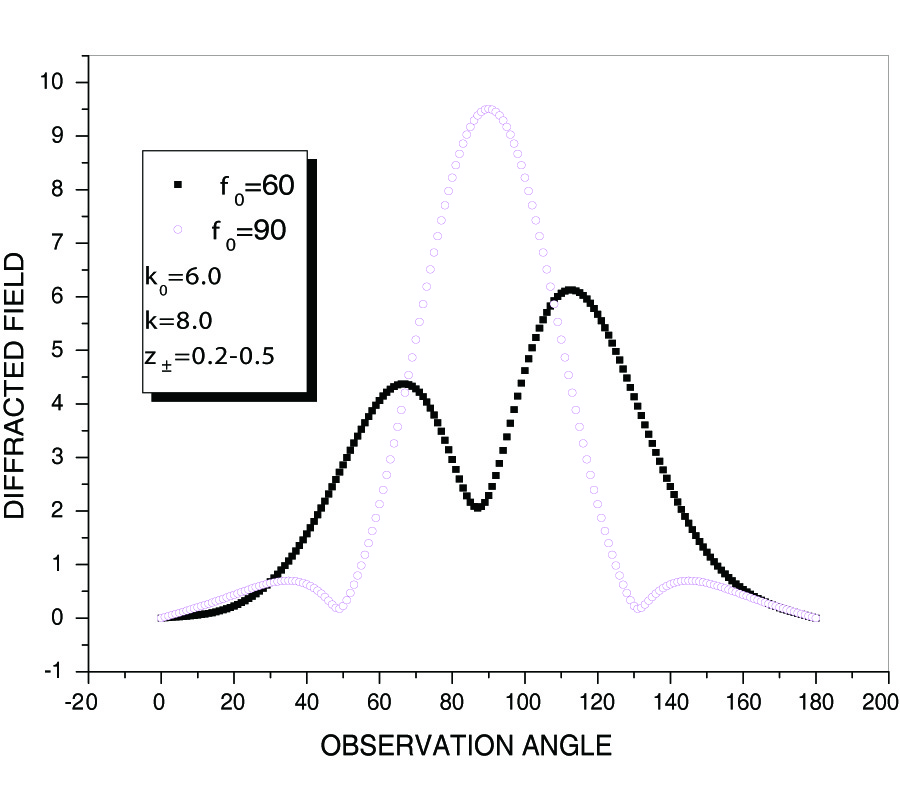# Vol. 10

Latest Volume
All Volumes
All Issues
2008-10-22

#### Diffraction from a Slit in an Impedance Plane Placed at the Interface of Two Semi-Infinite Half Spaces of Different Media

By Amjad Imran, Qaisar Naqvi, and Kohei Hongo
Progress In Electromagnetics Research B, Vol. 10, 191-209, 2008
doi:10.2528/PIERB08100802

## Abstract

Diffraction of an electromagnetic plane wave from a slit in an impedance plane placed at the interface of two different media, has been formulated rigorously. Both the principal polarizations are considered. The method of analysis is Kobayashi Potential (KP). To determine the unknown weighting functions, boundary conditions are imposed which resulted into dual integral equations (DIEs). These DIEs are solved by using the discontinuous properties of Weber- Schafheitlin's integrals. The resulting expressions are then expanded in terms of Jacobi's polynomials. The problems are then, reduced to matrix equations with infinite number of unknowns whose elements are expressed in terms of infinite integrals. These integrals are hard to solve analytically. The integrals contain poles for particular values of surface impedance and are solved numerically. Illustrative computations are given for far diffracted fields and other physical quantities of interest. To check the validity of our work, we compared the far field patterns with those of obtained through Physical Optics (PO). The agreement is good.

## Citation

Amjad Imran, Qaisar Naqvi, and Kohei Hongo, "Diffraction from a Slit in an Impedance Plane Placed at the Interface of Two Semi-Infinite Half Spaces of Different Media," Progress In Electromagnetics Research B, Vol. 10, 191-209, 2008.
doi:10.2528/PIERB08100802
http://test.jpier.org/PIERB/pier.php?paper=08100802

## References

1. Ziolkowski, R. W. and N. Engheta, "Metatamaterials," IEEE Trans. Antennas and Propagation, Vol. 51, No. 10, Oct. 2003.

2. Munk, B. A., Frequency Selective Surfaces: Theory and Design, John Wiley, 2000.

3. Hongo, K., "Diffraction of electromagnetic plane wave by a slit," Trans. Inst. Electronics and Comm. Engrg. in Japan, Vol. 55-B, No. 6, 328-330, 1972.

4. Hurd, R. A. and Y. Hayashi, "Low frequency scattering by a slit in a conducting plane," Radio Sci., Vol. 15, 1171-1178, 1980.
doi:10.1029/RS015i006p01171

5. Illahi, A., Q. A. Naqvi, and K. Hongo, "Scattering of dipole field by a finite and a finite impedance cylinder," Progress In Electromagnetics Research M, Vol. 1, 139-184, 2008.
doi:10.2528/PIERM08013001

6. Kobayashi, I., "Darstellung eines potentials in zylindrischen koordinaten, das sich auf einer ebene innerhalb und ausserhalb einer gewissen kreisbegrenzung verschiedener grenzbedingung unterwirft ," Sci. Rep. Tohoku Univ., Ser. 1/20, 197-212, 1931.

7. Sneddon, I. N., Mixed Boundary Value Problems in Potential Theory, North-Holland, Amsterdam, 1966.

8. Hongo, K. and H. Serizawa, "Diffraction of electromagnetic plane wave by a rectangular plate and a rectangular hole in the conducting plate," IEEE Trans. on Antennas and Propagation, Vol. 47, No. 6, 1029-1041, June 1999.
doi:10.1109/8.777128

9. Imran, A., Q. A. Naqvi, and K. Hongo, "Diffraction of plane wave by two parallel slits in an infinitely long impedance plane using the method of kobayashi potential," Progress In Electromagnetics Research, Vol. 63, 107-123, 2006.
doi:10.2528/PIER06042601

10. Hongo, K. and Q. A. Naqvi, "Diffraction of electromagnetic wave by disk and circular hole in a perfectly conducting plane," Progress In Electromagnetics Research, Vol. 68, 113-150, 2007.
doi:10.2528/PIER06073102

11. Serizawa, H. and K. Hongo, "Radiations from a flanged rectangular waveguidee," IEEE Tran. on Antennas and Propagation, Vol. 53, No. 12, 3953-3962, Dec. 2005.
doi:10.1109/TAP.2005.859748

12. Imran, A., Q. A. Naqvi, and K. Hongo, "Diffraction of electromagnetic plane wave by an strip," Progress In Electromagnetics Research, Vol. 75, 303-318, 2007.
doi:10.2528/PIER07053104

13. Naqvi, Q. A., K. Hongo, and H. Kobayashi, "Surface fields of an impedance wedge at skew incidence," Electromagnetics, Vol. 22, 209-233, 2002.
doi:10.1080/02726340252886474

14. Magnus, W., F. Oberhettinger, and R. P. Soni, Formulas and Theorems for the Special Functions of Mathematical Physics, Spinger-Verlag, New York, 1966.

15. Watson, G. N., A Treatise on the Theory of Bessel’s Functions, Cambridge University Press, 1944.